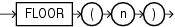FLOOR

SyntaxDescription of the illustration floor.eps

Purpose

FLOOR returns the largest integer equal to or less than n. The number n can always be written as the sum of an integer k and a positive fraction f such that 0 <= f < 1 and n = k + f. The value of FLOOR is the integer k. Thus, the value of FLOOR is n itself if and only if n is precisely an integer.

This function takes as an argument any numeric data type or any nonnumeric data type that can be implicitly converted to a numeric data type. The function returns the same data type as the numeric data type of the argument.